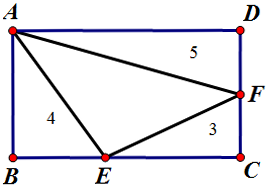# Fractions on a rectangle

Geometry Level 4

In the rectangle $ABCD$ below, $E$ lies on $BC$ and $F$ lies on $CD$ such that the area of 3 triangles $CEF$, $ABE$, $ADF$ are 3, 4 and 5, respectively.If $\dfrac {BE}{BC}+\dfrac{DF}{DC}= \dfrac ab$, where $a$ and $b$ are coprime positive integers, what is $a+b$?

×